# Geometry basic rules

By Martin McBride, 2022-04-30
Tags: angles in triangle angles in quadrilateral exterior angle angles on point angles around line isosceles triangle
Categories: gcse geometryHere are some basic rules and definitions of geometry that are useful to know. They are also covered in this video:

## Angles around a point

There are several geometry rules for angles.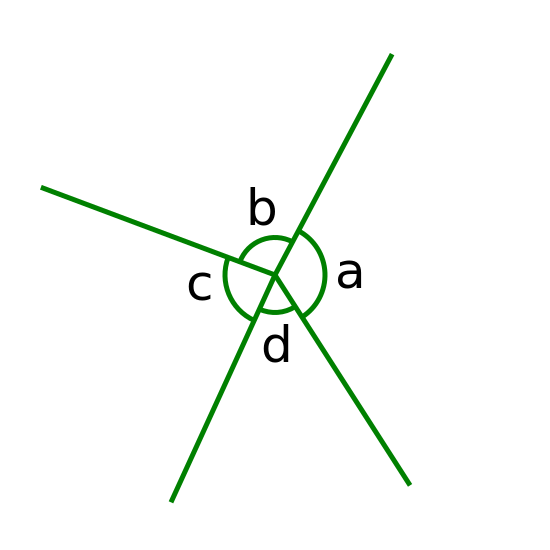The angles around a point add up to 360°.

a + b + c + d = 360°


## Angles on a line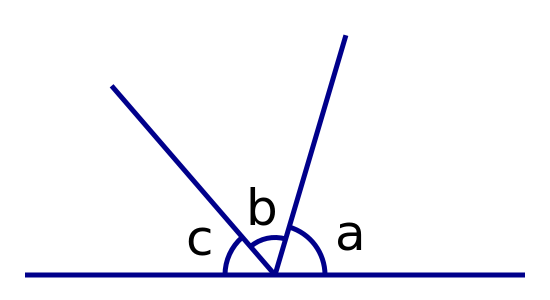The angles on a straight line add up to 180°.

a + b + c = 180°


## Angles in a triangle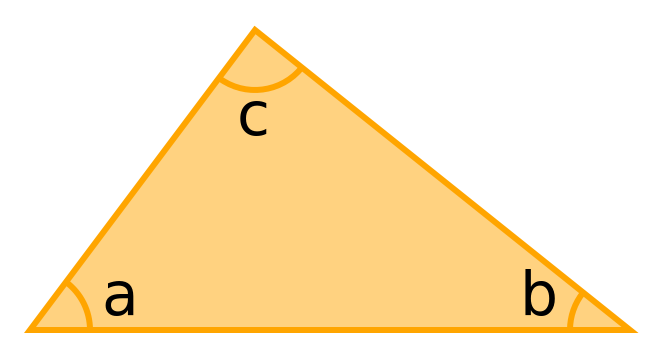The interior angles in a triangle add up to 180°.

a + b + c = 180°The interior angles in a quadrilateral (any 4-sided shape) add up to 360°.

a + b + c + d = 360°


## Exterior angles of a triangle

There are a number of geometry rules for triangles.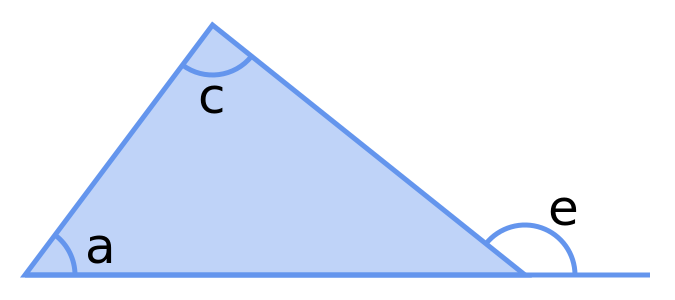An exterior angle of a triangle is equal to the sum of the 2 opposite interior angles.

e = a + b


## Angles and sides of an isosceles triangle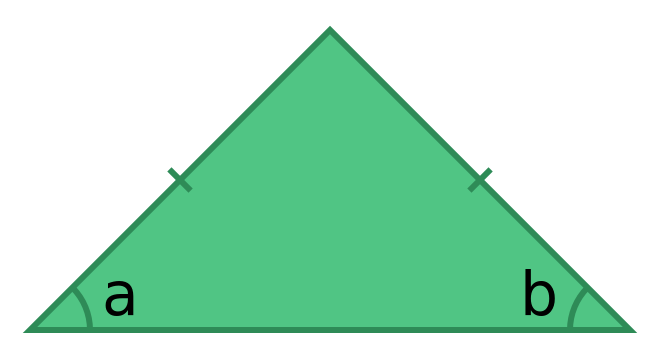An isosceles triangle has two sides of equal length (the two sides indicated by the tick marks that are drawn across the lines). It also has two equakl angles:

a = b